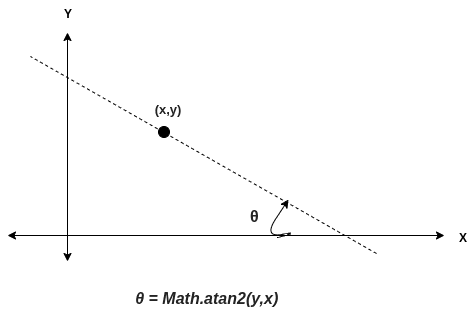# JavaScript Math atan2() Method

JavaScript Math atan2() is a built-in function that returns an arctangent of the quotient of its arguments. The atan2() method returns a numeric value between -Π and Π representing the angle theta of the (x, y) point and the positive x-axis.

To find the arctangent quotient of two passed arguments in JavaScript, use the Math.atan2() method.

See the following figure.Since atan2() is a static method of Math, it can be used without creating an object.

### Syntax

``Math.atan2(y,x) ``

### Parameter(s)

The variables y and x, whose arctangent quotient is to be determined.

### Return Value

The arctangent quotient is in the range from -pi to pi radians.

See the following figure.### Example 1

The following example demonstrates the use of the atan2() method.

``````var x = 5;
var y = 4;

console.log(Math.atan2(y, x));

var x = 90;
var y = 15;

console.log(Math.atan2(y, x));

var x = 15;
var y = 90;

console.log(Math.atan2(y, x));``````

Output

``````0.6747409422235527
0.16514867741462683
1.4056476493802699``````

### Example 2

The following example demonstrates the case where an empty value is passed.

``````var x;
var y;

console.log(Math.atan2(x, y));
console.log(Math.atan2());``````

Output

``````NaN
NaN``````

### Example 3

If the second parameter is passed as negative zero, and the first argument is ±0, the method returns pi with the same sign as the first argument.

``````var x = -0;
var a = 0;
var b = -0;

console.log(Math.atan2(a, x));
console.log(Math.atan2(b, x));``````

Output

``````3.141592653589793
-3.141592653589793``````

That’s it.

This site uses Akismet to reduce spam. Learn how your comment data is processed.